Question

Let a and b be the lengths of the legs of a right triangle, and c...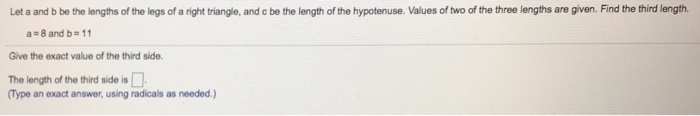Let a and b be the lengths of the legs of a right triangle, and c be the length of the hypotenuse. Values of two of the three lengths are given. Find the third length. a 8 and b 11 Give the exact value of the third side. The length of the third side is Type an exact answer, using radicals as needed.)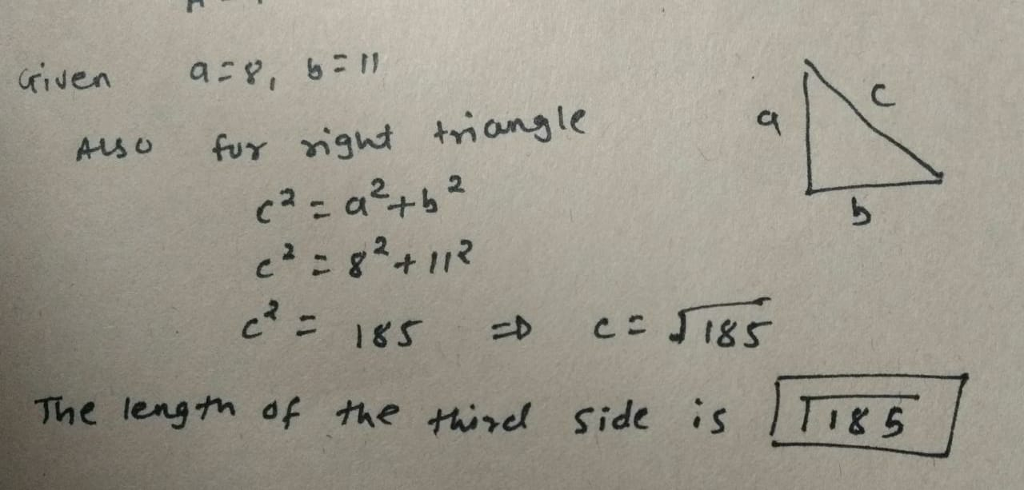Earn Coins

Coins can be redeemed for fabulous gifts.

Similar Homework Help Questions
• the Pythagorean theorem to find the length of the unknown side of a right triangle, where...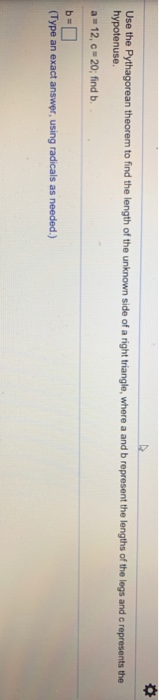the Pythagorean theorem to find the length of the unknown side of a right triangle, where a and b represent the lengths of the legs and c represents the hypotenuse. a 12, c 20, find b (Type an exact answer using radicals as needed.)

• The Pythagorean Theorem for right triangles states that if a and b are the lengths of the legs of a right triangle and c is the length of the hypotenuse, then a2 C b2 D c2

The Pythagorean Theorem for right triangles states that if a and b are thelengths of the legs of a right triangle and c is the length of the hypotenuse,then a2 C b2 D c2

• The lengths of 2 legs of a right triangle are 16 cm and 30 cm

The lengths of 2 legs of a right triangle are 16 cm and 30 cm. What is the length of the hypotenuse?

• core: 0 of 1 pt 70 of 77 (77 complete) 18.2.53 The hypotenuse of an isosceles...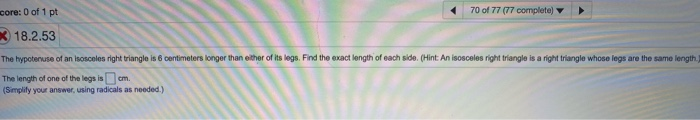core: 0 of 1 pt 70 of 77 (77 complete) 18.2.53 The hypotenuse of an isosceles right triangle is 6 centimeters longer than either of its legs. Find the exact length of each side. (Hint An isosceles right triangle is a right triangle whose legs are the same length. The length of one of the legs is (Simplify your answer using radicals as needed.) cm

• If the hypotenuse of a right triangle is 13 and one of its legs is 5, find the area fo the triangle

If the hypotenuse of a right triangle is 13 and one of its legs is 5, find the area fo the triangle.Answer Choices2040453025I know a hypotenuse the side of a right triangle opposite the right angle. You can't multiply the hypotenuse with one of the legs.

• In a right triangle, a and b represent legs and c represents the hypotenuse. Determine the...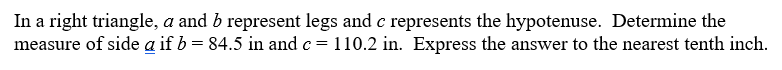In a right triangle, a and b represent legs and c represents the hypotenuse. Determine the measure of side a if b = 84.5 in and c= 110.2 in. Express the answer to the nearest tenth inch.

• 3.11.13-Setup & Solve The legs of an isosceles right triangle increase in length at a rate...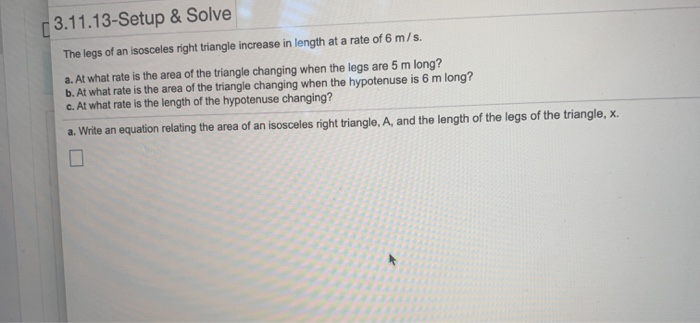3.11.13-Setup & Solve The legs of an isosceles right triangle increase in length at a rate of 6 m/s. a. At what rate is the area of the triangle changing when the legs are 5 m long? b. At what rate is the area of the triangle changing when the hypotenuse is 6 m long? c. At what rate is the length of the hypotenuse changing? a. Write an equation relating the area of an isosceles right triangle, A, and...

• Find the length of the hypotenuse of an isosceles right triangle whose legs are 1 unit in length

Find the length of the hypotenuse of an isosceles right triangle whose legs are 1 unit in length.

• The hypotenuse of a right angle triangle is 3 meters longer than twice the length of one of the legs

The hypotenuse of a right angle triangle is 3 meters longer than twice the length of one of the legs. The measure of the other leg is 12 meters. Find the measure of the hypotenuse of the triangle.

• find the hypotenuse of a right triangle with side lengths of 7 and 0. round answer...

find the hypotenuse of a right triangle with side lengths of 7 and 0. round answer one decimal place/